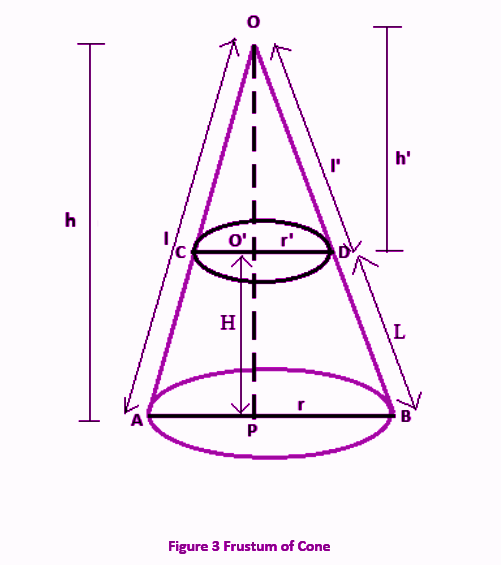# Volume of a Frustum

The volume of a frustum of a cone depends on its slant height and radius of the upper and bottom circular part. Basically a frustum of a cone is formed when we cut a right-circular cone by a plane parallel to its base into two parts. Hence, this part of the cone has its surface area and volume.

 Volume of frustum of cone = πh/3 (r12+r22+r1r2)

Let us learn here to derive the volume of frustum and understand the concept better by solving the problems.

## Derivation of Volume of Frustum of Cone

Let us consider a right circular cone which is cut by a plane parallel to its base as given in the below figure.Here, we can consider the frustum as the difference between the two right circular cones.

Let,

Height of large cone = h

Slant height of large cone = l

Now,

Height of smaller cone = h’

Slant height of smaller cone = l’

In case of frustum,

Height of frustum = H

Slant height= L

Now, we can say,

volume of bigger cone say V1 = ⅓ π r2h

Also, volume of smaller cone, V2 = ⅓ πr’2h’

Therefore,

Volume of frustum of cone, V = V1 – V2

V = ⅓ π r2h – ⅓ πr’2h’

V = ⅓ π (r2h – r’2h’) ……………(1)

From the figure, in the ∆OO’D and ∆OPB;

∠DOO’ = ∠BOP (Common Angle)

CD//AB (Plane cutting the cone is parallel to the base)

⇒∠O’DO = ∠PBO (Corresponding Angles)

Thus, ∆OO’D~∆OPD (By AA criterion of similarity)

As we know, by the condition of similarity, the ratio of sides of similar triangles are equal. Hence,

h’/h = r’/r …………………..(2)

Substituting the value of eq. 2 in eq. 1 we get;,

$$V$$ = $$\frac{1}{3}~ π\left(r^2 \left(\frac{rh’}{r’}\right)~-~r’^2 h’\right)$$ $$⇒~V$$ = $$\frac{1}{3}~ πh’~ \left(\frac{r^3~-~r’^3}{r’}\right)$$ …………(3)

From the figure we can see;

h = H + h′

Substituting this value in equation (2), we get;

$$\frac{h’}{H~+~h’}$$ = $$\frac{r’}{r}$$ $$⇒~\frac{h’}{H}$$ = $$\frac{r’}{r~-~r’}$$ $$⇒~h'$$ = $$H\left(\frac{r’}{r~-~r’}\right)$$

Now putting the value of h’ in equation 3 we get;

$$V=\frac{1}{3}\pi H(\frac{r{‘}}{r-r{‘}})(\frac{r^3-r{‘}^3}{r{‘}})$$ $$V=\frac{1}{3}\pi H(\frac{r-r{‘}}{r-r{‘}})({r^2+rr{‘}+r{‘}^2})$$ $$⇒~V$$ = $$\frac{1}{3}~ πH~(r^2~+~r’r~+~r’^2)$$

In few cases, we take,

r = R or r1 and r’ = r or r2

If radius of base of frustum is R and top of frustum is r, then the volume can be represented by:

Volume of frustum of cone = πh/3 (R2+r2+Rr)

If radius of base of frustum is r1 and top of frustum is r2 then the volume can be represented by:

Volume of frustum of cone = πh/3 (r12+r22+r1r2)

### Slant Height of Frustum of Cone

The slant height of frustum of cone is determined by the formula:

Slant Height = √[(r1 – r2)2 + h2]

The slant height of frustum plays an important role while determining its lateral surface area.

## Problem and Solution

If the radius of base of cone is 20 m and radius of cone obtained after we have cut it by a plane is 8 m, and height of frustum of cone is 30 m, then find the volume of frustum.

Solution: Given,

R = 20 m

Base of small cone obtained will be the top of frustum.

Therefore, r = 8 m

Height of frustum = 30 m

By the formula of volume we know;

Volume of frustum of cone = πh/3 (R2+r2+Rr)

V = π(30)/3 (202+82+20×8)

V = 19611.42 cubic meter

## Frequently Asked Questions on volume of a frustum

### What is the formula for volume of frustum of cone?

Volume of frustum of cone = πh/3 (R2+r2+Rr)

Where R is the radius of bottom surface, r is the radius of top surface and h is the height of frustum.

### How to find the volume of frustum of cone?

To find the volume of frustum, we can find the volume of larger cone first and subtract the volume of smaller cone obtained, when we cut the right circular cone by a plane horizontally. The plane which cuts the cone should be parallel to the base.

### What is the frustum of cone?

When a cone is cut by a plane horizontally, parallel to the base of the cone, then the lower part of the cone is called a frustum.

### What is the total surface area of frustum?

The total surface area of frustum is given by;

Surface area = π x [R2 + r2 + (R + r) x √((R – r)2 + h2)]

### What is CSA of a frustum?

The curved surface area of frustum of a cone is given by;

CSA = πl(R – r)

Where r is the radius of upper circle or small circle of frustum and R is the radius large circle or base and l is the slant height.

Test your Knowledge on Volume of a Frustum